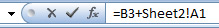# With the increasing development of mathematics and applications of this field and the frequency of the number of formulas and the complexity of some formulas,

to accurately calculate these formulas and prevent errors, we can use excel software.

## Excel Formulas:

Formulas are a set of mathematical instructions used to perform calculations in this program. Formulas in the formula bar or Excel cells that you want (destination house) start with the = sign, then we enter the desired formula in the destination cell.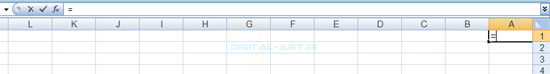To create a basic formula in Excel, you must start by placing an equal sign in the Excel cell in which the result of the calculation is written (destination house) and select the cells to be used in this formula, then the formula in the house. Destination is displayed ..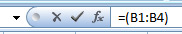## Consecutive and non-consecutive cells:

Cells are selected sequentially and non-sequentially to determine the formulation range:

## Consecutive:

Several consecutive cells (in a row) are selected for the formula, which is marked with an asterisk (:) between the cell names. For example, if we select from cell B1 to B4, it is written as (B1: B4) = and indicates the selection of consecutive cells for the formula.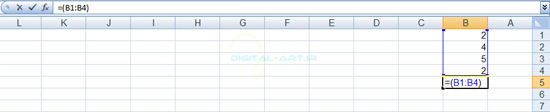## Invalidity:

If we select several cells that are located in different places (they are not in a row), we will see a sign (;) in the name of the selected cells, which indicates the selection of non-consecutive cells written in the formula. For example, if we select only cells B1 and B3 to write the formula, the formula is written as (B1; B3) =. For example, click here on cell B5, which is intended as the destination cell. An equal sign is then placed in this cell. Then select from formula B1 to B4 by dragging (data in a row). The result of the operation in cell B5 will be as follows (B1: B4) = that this is a formula written in Excel and there is no answer in action and after pressing the Enter key, you will encounter the answer (incorrect Value).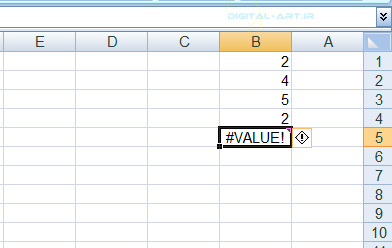– Calculate with Operators (Calculate with Operators)

To calculate the data in Excel, the operation of computational groups (+, -, *, /, etc.) is used, which are also the most important part of the calculation. The table below shows the 6 main operations in mathematics.Formula writing steps in Excel:

1- First, put the equal sign (=) in the desired cell and write the numbers and the mathematical calculator, respectively. For example, in the figure below, I performed the subtraction operation in cell B1. (The formula is written as 350-500 = the answer is 150)

• If the calculation is in a cell and the answer is in the same cell:

### To calculate non-consecutive data:

We are going to do a multiplication operation here. To calculate the data and put the result in the destination, first put an equal sign in the destination house and then open the parentheses. Now select the cells in which the data is located. For example, select cells B1 and B4. After selecting the first cell (B1), put a multiplication symbol (*), then select the second cell by pressing Ctrl.

In the destination cell, a formula will be written as follows.

(B1 * B4) =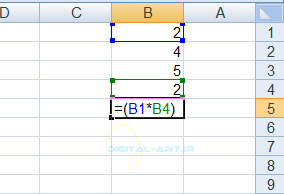Now press the Enter key on the keyboard to perform the required operation and write the result in the destination cell. The result is multiplied by (4).

• Note: The above formula is used for all four basic mathematical operations.

Collect a number of consecutive cells:

To do this, first put an equal sign (=) in the destination cell where the answer should be placed, then type Sum and drag to select the desired cells.

In the following example, add cells B1 to B4 together, the formula of which will be (Sum (B1: B4 =), and by pressing the Enter key on the keyboard, I have reached (13) in the destination cell (B5).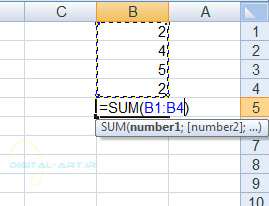Note: If you want to multiply the cells in a row, use the word Product instead of the word Sum and write the formula as before.

In the example, cells B1 to B4 are multiplied, the formula of which is written as Product (B1: B4 = and the result in cell B5 will be equal to (80)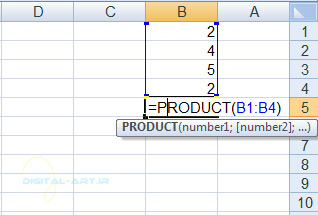Note: Changing the data of each cell that has been added or multiplied consecutively will change the answer you wrote in the destination cell.

Example: If we change the data of cell B2 to 8, you will see that after pressing the Enter key, the answer written in cell B5 changes to (160).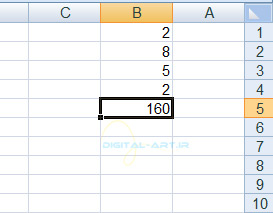## Addition of a number of non-sequential cells:

To do this, first put an equal sign (=) in the desired cell. Then write the word Sum and after opening the parentheses and pressing the Ctrl key to select the desired cells. Then press the Enter key to see the answer in the destination cell.

## Example:

I want to add cells B1, B3 and B4, which are incomplete, and put the answer in cell B5. First we put an equal sign in the destination cell (B5) and then write the word Sum and by opening the parentheses I select these three cells and then close the parentheses. The formula will be written as (Sum (B1; B3; B4 =) and will be written in cell B5 by pressing the Enter key whose answer is (9).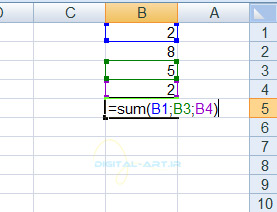## Very important point:

In calculations and formula writing, all calculations are done from the left, and this is a rule in mathematics. But in Excel program, prioritization is defined for performing calculations and using mathematical operators, and the program calculates the data according to this prioritization. This is the prioritization.

## for example:

If the formula is written as 4 * 2 + 10 =, the result of the calculation will be 18 because according to the priority of the calculation in Excel, first the operator numbers are multiplied and then the sum. But if we write the formula with 4 * (10 + 2) = prioritization, first the numbers 2 and 10 are added together (because the parentheses have a higher precedence) then it will be multiplied by the number 4, so the result will be 48 correctly. .

3- Calculate with Functions

## There are two ways to access the functions:

The first way:

From the Home tab, go to the Editing box and click on the small arrow next to the option shown (Auto Sum) in the image below to open the functions box.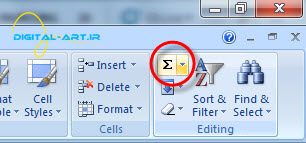The second way:

Go to the Formulas tab and in the Function Library box, click on the small arrow next to the Auto Sum option to open the functions in the box below this option.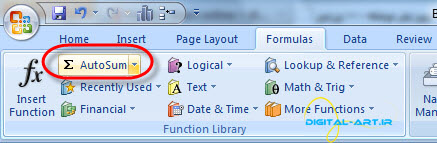Functions shown in the sum option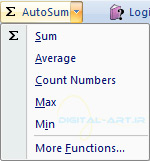## Sum (sum operation):

Specify the destination cell first. Then by clicking on this option, you will see that the word Sum is written in the desired house. Now it is enough to drag the range of selected cells to apply the addition operation on them.

## Average:

First, specify the destination cell and by selecting this option, you will see that the word Average is written in the target cell. Now it is enough to select the desired cell range or cells to calculate the average of these cells.

## Count (numerical cell count):

First specify the destination cell. Then by clicking on this option, you will see that the word Count is written in the destination cell. Now it is enough to select the desired cells to perform the cell count operation on them.

It should be noted that in this method, empty cells and cells that contain text are not counted.

## Min (find the minimum value):

First specify the desired cell and by selecting this option you will see that the word (minimum value) Min is written in the destination cell. Now it is enough to drag the selected cells and write the least data in these cells in the destination cell .

## Max (find the maximum value):

First specify the destination cell and by selecting this option you will see that the word (maximum value) Max is written in the destination cell. Now it is enough to select the desired cell range to write the most data in these cells in the selected cell .

## 4- Function Library

The function library has a wide range of functions. To see the Function Library, go to the Formula tab from the toolbar at the top of the program. On the left side of this tab you will find a group of functions called Function Library which has many options. These functions are as follows.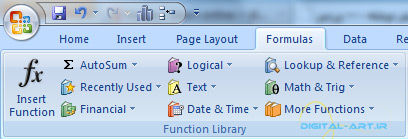## Auto and fast aggregator (AutoSum):

Easily calculates selected cellular data.

Recently Used Functions: Specifies all the functions you have recently used.

## Financial:

Will calculate financial data.

Logical: Specifies true, false and..

Text: Functions that are text-based.

Date & Time: will calculate date and time functions.

Mathematics and Trigonometry (Math & Trig): which has mathematical functions and these functions can be used in work.

Note: All these functions have a subset that by clicking on the small arrow to their right, select the required function from the opened box.

5. Relative, Absolute, & Mixed Functions

In Excel, cellular addresses are written and used in two ways:

In this type of addressing, the position of the cell relative to the cell in which the formula is written is shown. For example, address B3 is a relative address, and in writing a relative address, first the name of the column and then the name of the row are written.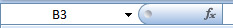This type of addressing specifies the absolute position of a cell. To write this type of address, the dollar sign (\$) is used before the name of the column and row. For example, the absolute address that will be formed from the collision of column b and row 3 would be like this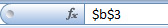## Copy formula:

We will first use the relative address in the formula: Suppose in the table below you want to get the total price for each product. The total price value is equal to the product number multiplied by its unit price. First, go to cell E2 and write its formula as D2 * C2 =. To make things easier in terms of formula writing in column E, after writing the formula in cell E2, you can use the Auto Fill feature (described in step three) and fill cells E3 to E7 by copying this formula. Multiplication will be different in the target cells.

Note: Because we have used the relative address in formula E2, after copying this formula in the cell, for example E3, its formula will change to D3 * C3 =, the formula of which will be written in the formula bar as follows: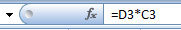As the name implies, this type of addressing is absolute and will not change anything. Note that sometimes the result of your calculations is determined by the type of addressing. Sometimes in formula writing, it is necessary to write one of the row or column addresses in absolute terms and the other in relative terms. This type of addressing is called hybrid addressing. Like A \$ 1 where column A is entered partially and row 1 is entered absolutely. To convert the relative and absolute addresses of a cell, just press the F4 key on your keyboard to convert the address of a cell to the types mentioned above. Like the following example: